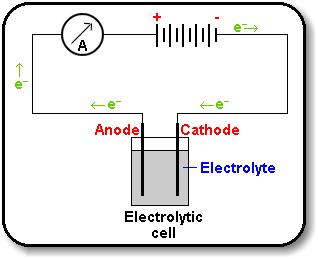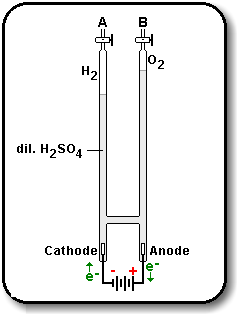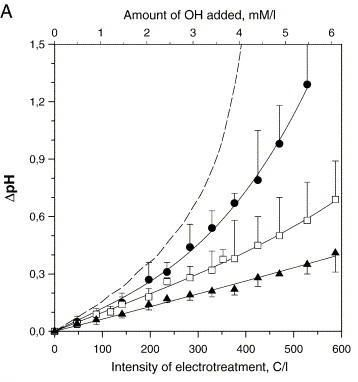Changing pH Using Electrolysis

## Introduction

Electrolysis is a process that takes place in an electrolytic cell. An example of the cell is illustrated below:The electrodes are the parts which make contact with the electrolyte. The cathode is the electrode which is connected to the negative pole of the battery, which supplies the electrons to the electrolyte. On the other hand, the anode is the electrode which is connected to the positive pole of the battery, which accepts the electrons to the electrolyte.

The process that takes place at the cathode is called reduction, and the process that takes place at the anode is called oxidation. So when a direct current is passed through an electrolyte such as aqueous solution of a salt, acid or base, chemical reactions take place at the contacts between the circuit and solution. This process is called electrolysis.

## Electrolysis of Water

Pure distilled water is a poor conductor of electricity because the small numbers of H+ and OH- ions (10-7 mol/dm³). Hence dilute sulphuric acid must be added in order to have a significant current flow between the electrodes. Below is a setup of how electrolysis of water can be done:The process that takes place at the cathode (positive pole) is the reduction of protons:

4H2O + 4e- = 4H+ + 4OH- + 4e- -> 2H2(g) + 4OH- = -0.83 v

The process that takes place at the anode (negative pole) is the oxidation. In this case, there are two anions competing to give up their electrons from the ionization of water. They are sulphate (SO42-), and hydroxide (OH-). The oxidation of OH- has a standard electrode potential of -0.40V, as compared to the oxidation of sulphate which is -0.17V. Hence OH- is preferred. The process equation is as follow:

2H2O = 2H+ + 2OH- ->O2 + 4H+ + 4e- = -1.23 v

Hence the overall equation can be written as:

2H2O -> 2H2 + O2 = -2.06 v

From the equation above, if a potential of greater than 2.06V is applied, this reaction will take place.

Similarly, if the solution Sodium Hydroxide is used, there will be two cations competing to receive the electrons, namely H+ and Na+. In this case, H+ will be preferred.

## Changing pH using Electrolysis

From the two examples above, we can see that it is not possible to change the concentration of H+ without changing the concentration of OH- at low voltage. Hence it is not possible to change the pH from an acidic solution to neutral or from basic solution to neutral at low voltage.

“From the figure below, it can be seen that under moderate electric treatment, the change of pH is not significant.” Hence in order to change the pH of a solution, high voltage is required.## Disadvantages of using Electrolysis to change pH

1) Change of pH is difficult to control when it crosses the neutral point.
2) Hydrogen gas, which is a highly flammable gas, is a by product of the process.
3) High Voltage used during the process might destroy the plant.

## Reference

 Saulis G, Lape R, Praneviciute R, Mickevicius D, Changes of the solution pH due to exposure by high-voltage electric pulses. Bioelectrochemistry 67 (1): 101-108 Sep 2005.

page revision: 10, last edited: 20 Sep 2007 18:57